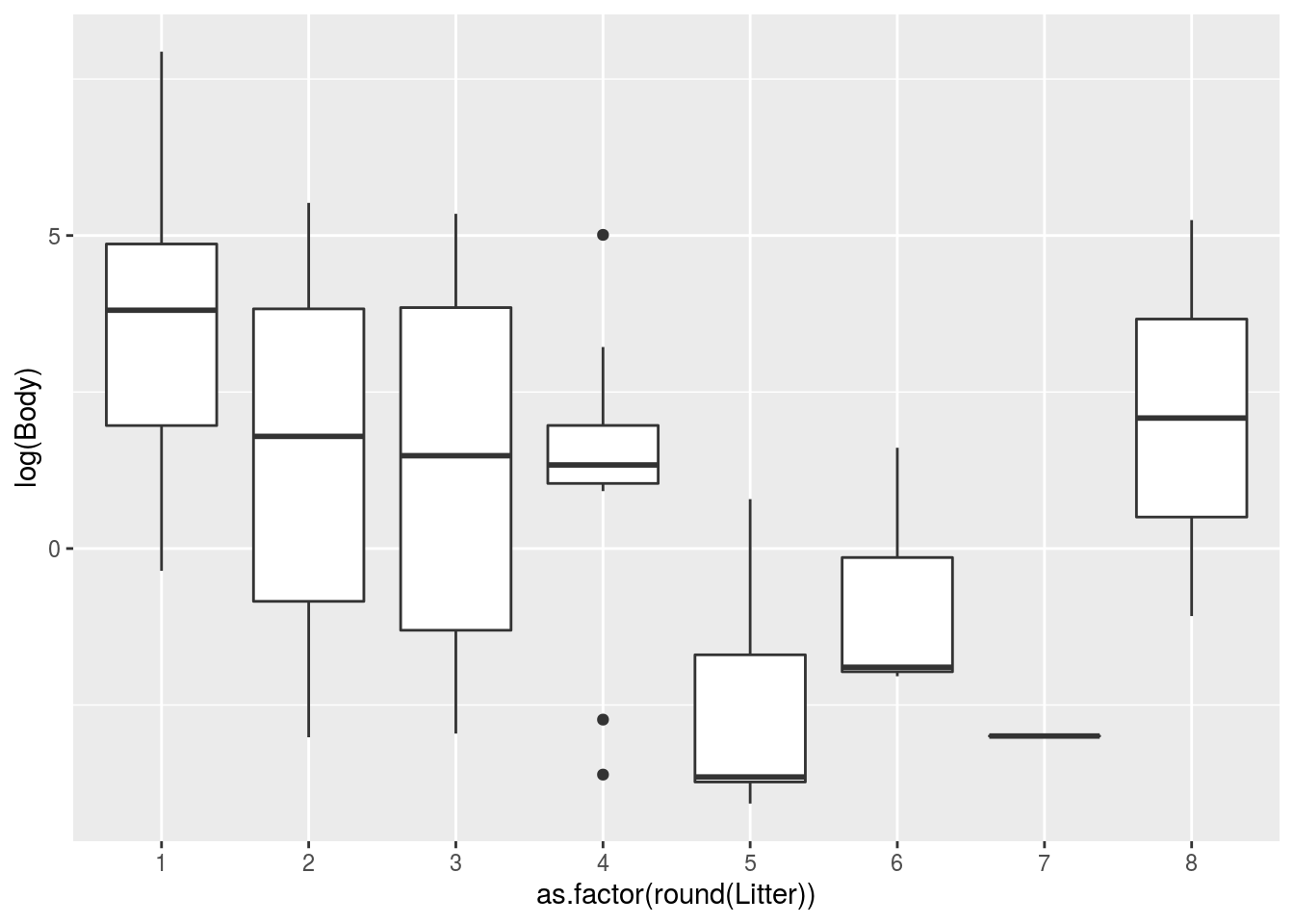Run the following to load a dataset that records various data about mammals, including brain weight. The brain weight is given in grams, the body weight in kilograms, and the gestation weight in days.

brains <- read.csv("http://guerzhoy.princeton.edu/201s20/brains.csv")

### Problem 1: Linear Regression

#### Part 1(a)

Suppose you want to use linear regression to investigate the relationship between brain weight and body weight. Find a way to transform the variables that would allow you to do that. (Hint: try taking the log of variables). Use a scatterplot to assess whether a relationship is linear.

#### Part 1(b)

Produce the diagnostic plots for the linear fit you found in Part 1(a) (use autoplot). Display and investigate outliers, if any. (See Tuesday’s lecture on the relationship between gdp per capita and life expectancy)

#### Part 1(c)

Display the summary for the regression. What conclusions can you draw?

### Problem 2: Failing to meet assumptions

Suppose we want to know whether the size of the litter is related to body weight. Produce diagnostic plots for any variable transformations you can think of. Do not expect the linear regression model assumption to hold.

### Problem 3: Litter size as categorical

Treat rounded average litter size as categorical (you will need to convert litter size to factor or character). Plot the appropriate diagnostics. Are the model assumptions satisfied? Use a boxplot to assess whether the model assumptions hold. Reminder: you are looking for approximately constant variance.

#### Solution (since we didn’t cover quite cover model assumptions)

Basically, we need for the distribution around each mean to be Gaussian, since what we’re doing is predicting with a categorical variable. That makes sure that the residuals are Gaussian

library(tidyverse)
ggplot(brains) +
geom_boxplot(mapping = aes(y = log(Body), x = as.factor(round(Litter))))Those are not generally symmetrical and the variance of the residuals is not constant.

### Problem 4: Litter size as ever more categorical

Create a new variable: litter size is greater than 5. Check the model assumptions. Now, use lm to test the hypothesis that the body weight is related to the litter size being greater than 5. What conclusions can you draw?

Make sure to transform any variables that need to be transformed for the model assumptions to hold.

### Problem 5: the F-test

Create another new variable with the categories: litter size up to 2, litter size up 7, litter over 7. Produce appropriate diagnostic plots, and use an F-test to compute a p-value. What is the null hypothesis? What is the conclusion?

Make sure to transform any variables that need to be transformed for the model assumptions to hold.

The following code might be useful:

a <- c(1, 20, 100, 10000, 1)
b <- rep("small", length(a))
b[a > 10] <- "medium"
b[a> 150] <- "large"
b
##  "small"  "medium" "medium" "large"  "small"